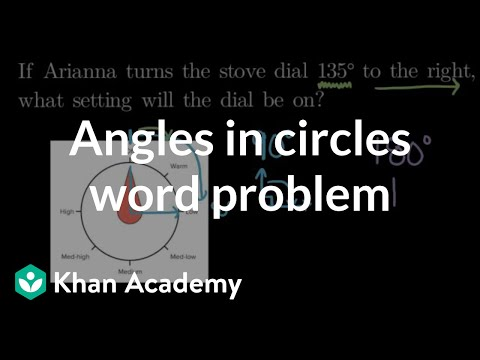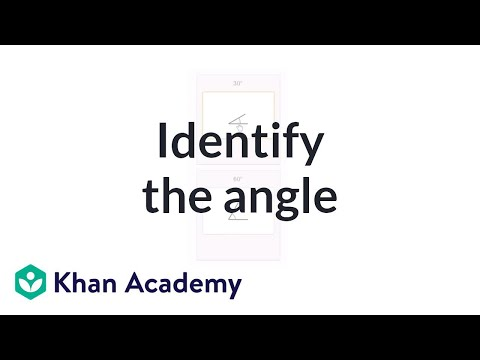Video

# Angle measurement & circle arcs (Full video)

Description: Learn to measure angles as part of a circle. Created by Sal Khan. We already know that an angle is formed when two rays share a common endpoint. So, for example, let's say that this is one ray right over here, and then this is one another ray right over here, and then they would form an angle. And at this point right over here, their common endpoint is called the vertex of that angle.

### Other videos you might be interested in### Angles in circles word problem (Full video)